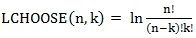# SQL Server natural logarithm of the binomial coefficient function

LCHOOSE

Updated: 30 September 2015

Use LCHOOSE to calculate the natural logarithm of the binomial coefficient.Syntax
SELECT [wct].[LCHOOSE](
<@N, float,>
,<@K, float,>)
Arguments
@N
is any positive number. @N must be of a type float or of type that intrinsically converts to float
@K
is any positive number. @K must be of a type float or of a type that intrinsically converts to float.
Return Type
float
Remarks
·         @N is truncated and the integer value is used.
·         @K is truncated and the integer value is used.
·         If @N < 0 then NULL is returned.
·         If @K < 0 then NULL is returned.
·         If @K > @N then NULL is returned.
Examples
SELECT
wct.LCHOOSE(
25    --@N
,15    --@k
) as LCHOOSE
This produces the following result.
Using the EXP function with LCHOOSE will return the binomial coefficient.
SELECT
EXP(wct.LCHOOSE(25,15)) as LCHOOSE,
wct.BICO(25,15) as BICO
This produces the following result.

### SupportCopyright 2008-2021 Westclintech LLC         Privacy Policy        Terms of Service